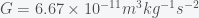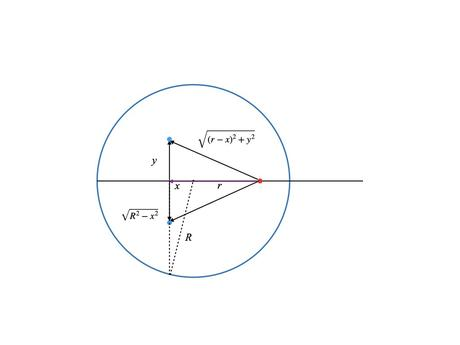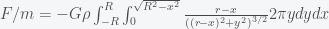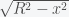# Falling Through the Earth

Posted on the 28 May 2022 by Ccc1685 @ccc1685

The 2012 remake of the classic film Total Recall features a giant elevator that plunges through the earth from Australia to England. This trip is called the "fall", which I presume to mean it is propelled by gravity alone in an evacuated tube. The film states that the trip takes 17 minutes (I don't remember if this is to get to the center of the earth or the other side). It also made some goofy point that the seats flip around in the vehicle when you cross the center because gravity reverses. This makes no sense because when you fall you are weightless and if you are strapped in, what difference does it make what direction you are in. In any case, I was still curious to know if 17 minutes was remotely accurate and the privilege of a physics education is that one is given the tools to calculate the transit time through the earth due to gravity.

The first thing to do is to make an order of magnitude estimate to see if the time is even in the ballpark. For this you only need middle school physics. The gravitational acceleration for a mass at the surface of the earth is. The radius of the earth is 6.371 million metres. Using the formula that distance(which you get by integrating twice over time), you get. Plugging in the numbers gives 1140 seconds or 19 minutes. So it would take 19 minutes to get to the center of the earth if you constantly accelerated at. It would take the same amount of time to get back to the surface. Given that the gravitational acceleration at the surface should be an upper bound, the real transit time should be slower. I don't know who they consulted but 17 minutes is not too far off.

We can calculate a more accurate time by including the effect of the gravitational force changing as you transit through the earth but this will require calculus. It's a beautiful calculation so I'll show it here. Newton's law for the gravitational force between a point mass m and a point mass M separated by a distance r iswhereis the gravitational constant. If we assume that mass M (i.e. earth) is fixed then Newton's 2nd law of motion for the mass m is given by. The equivalence of inertial mass and gravitational mass means you can divide m from both sides. So, if a mass m were outside of the earth, then it would accelerate towards the earth asand this number is g when r is the radius of the earth. This is the reason that all objects fall with the same acceleration, apocryphally validated by Galileo on the Tower of Pisa. (It is also the basis of the theory of general relativity).

However, the earth is not a point but an extended ball where each point of the ball exerts a gravitational force on any mass inside or outside of the ball. (Nothing can shield gravity). Thus to compute the force acting on a particle we need to integrate over the contributions of each point inside the earth. Assume that the density of the earthis constant so the masswhereis the radius of the earth. The force between two point particles acts in a straight line between the two points. Thus for an extended object like the earth, each point in it will exert a force on a given particle in a different direction. So the calculation involves integrating over vectors. This is greatly simplified because a ball is highly symmetric. Consider the figure below, which is a cross section of the earth sliced down the middle.The particle/elevator is the red dot, which is located a distance r from the center. (It can be inside or outside of the earth). We will assume that it travels on an axis through the center of the earth. We want to compute the net gravitational force on it from each point in the earth along this central axis. All distances (positive and negative) are measured with respect to the center of the earth. The blue dot is a point inside the earth with coordinates (x,y). There is also the third coordinate coming out of the page but we will not need it. For each blue point on one side of the earth there is another point diametrically opposed to it. The forces exerted by the two blue points on the red point are symmetrical. Their contributions in the y direction are exactly opposite and cancel leaving the net force only along the x axis. In fact there is an entire circle of points with radius y (orthogonal to the page) around the central axis where each point on the circle combines with a partner point on the opposite side to yield a force only along the x axis. Thus to compute the net force on the elevator we just need to integrate the contribution from concentric rings over the volume earth. This reduces an integral over three dimensions to just two.

The magnitude of the force (density) between the blue and red dot is given byTo get the component of the force along the x direction we need to multiple by the cosine of the angle between the central axis and the blue dot, which is(i.e. ratio of the adjacent to the hypotenuse of the relevant triangle). Now, to capture the contributions for all the pairs on the circle we multiple by the circumference which is. Putting this together givesThe y integral extends from zero to the edge of the earth, which is. (This is R at(the center) and zero at(the poles) as expected). The x integral extends from one pole to the other, hence -R to R. Completing the y integral gives(*)

The second term comes from the 0 limit of the integral, which is. The square root of a number has positive and negative roots but the denominator here is a distance and thus is always a positive quantity and thus must include the absolute value. The first term of the above integral can be completed straightforwardly (I'll leave it as an exercise) but the second term must be handled with care because r-x can change sign depending on whether r is greater or less than x. For a particle outside of the earth r-x is always positive and we getInside the earth, we must break the integral up into two partsThe first term of (*) integrates toUsing the fact that, we get(We again need the absolute value sign). For, the particle is outside of the earth) and. Putting everything together givesThus, we have explicitly shown that the gravitational force exerted by a uniform ball is equivalent to concentrating all the mass in the center. This formula is true fortoo.

Forwe haveRemarkably, the gravitational force on a particle inside the earth is just the force on the surface scaled by the ratio r/R. The equation of motion of the elevator is thuswith(Recall that the gravitational acceleration at the surface is). This is the classic equation for a harmonic oscillator with solutions of the form. Thus, a period (i.e. round trip) is given by. Plugging in the numbers gives 5062 seconds or 84 minutes. A transit through the earth once would be half that at 42 minutes and the time to fall to the center of the earth would be 21 minutes, which I find surprisingly close to the back of the envelope estimate.

Now Australia is not exactly antipodal to England so the tube in the movie did not go directly through the center, which would make the calculation much harder. This would be a shorter distance but the gravitational force would be at an angle to the tube so there would be less acceleration and something would need to keep the elevator from rubbing against the walls (wheels or magnetic levitation). I actually don't know if it would take a shorter or longer time than going through the center. If you calculate it, please let me know.

See more